# Use This, Not That

First create some utility functions and data for the benchmarks below

``````library(ggplot2)
library(microbenchmark)
library(knitr)
set.seed(20171130)

#-------------------------------------------------------------------------------
# Check for equality of results in a microbenchmark run
#-------------------------------------------------------------------------------
check_equality <- function(values) {
all(sapply(values[-1], function(x) identical(values[], x)))
}

#-------------------------------------------------------------------------------
# Convert multiple microbenchmark runs into a summarized data.frame.
#-------------------------------------------------------------------------------
## data_list A named list of data that is used in func_list.
## func_list An alist object of functions to be tested.
## size A vector of integers that represent the data size.
## times An integer of the number of microbenchmark runs.
## unit A character for time units of the microbenchmark runs.
## check TRUE or FALSE whether or not to verify output is identical in the
## microbenchmark run.
mb_summary <- function(data_list, func_list, size, times = 30, unit = "relative", check = TRUE) {
# Check arguments
list2env(data_list, envir = environment())

if(check) {
check <- function(values) {
all(sapply(values[-1], function(x) identical(values[], x)))
}
} else {
check <- NULL
}

# Run benchmarks
mb_results <- lapply(seq_along(size), function(x) {
microbenchmark::microbenchmark(
list = func_list,
times = times,
unit = unit,
check = check
)
})

# Create summary data.frame
summary_results <- do.call(
"rbind",
lapply(seq_along(mb_results), function(x) {
data.frame(
size = size[x],
summary(mb_results[[x]])
)
})
)

# return
summary_results[c("size", "expr", "lq", "median", "uq", "neval")]
}

#-------------------------------------------------------------------------------
# Time conversions relative to 1 second (fractions of a second)
#-------------------------------------------------------------------------------
time_conversion <- function(time) {
time_table <- data.frame(
value = c(120, 60, 1, 10^-(1:9)),
name = c(
"min",
rep("sec", each = 2),
rep("ms", each = 3),
rep("us", each = 3),
rep("ns", each = 3)
),
conversion = c(
1/60,
rep(1, 2),
rep(1000, 3),
rep(10^6, 3),
rep(10^9, 3)
),
stringsAsFactors = FALSE
)
closest_val = function(x,y){
diff = y - x
diff[diff <= 0] = NA
if(all(is.na(diff))) {
NA
} else {
which.min(diff)
}
}
units <- time_table\$name[sapply(time, closest_val, time_table\$value)]
convert <- time_table\$conversion[sapply(time, closest_val, time_table\$value)]
paste(signif(time*convert, 3), units)
}

#-------------------------------------------------------------------------------
# Create a vector for the log scale times that will look "pretty" on the y-axis.
#-------------------------------------------------------------------------------
## df A data.frame as returned by mb_summary().
## n_ticks An integer as the suggestion for the number of tick marks.
log_breaks <- function(df, n_ticks = 5) {
grDevices::axisTicks(
usr = log(range(df\$median, na.rm = TRUE), 10),
log = TRUE,
nint = n_ticks
)
}

#-------------------------------------------------------------------------------
# Determine the units of a microbenchmark.
#-------------------------------------------------------------------------------
mb_units <- function(mb) {
unit <- attr(mb, "unit")
if(is.null(unit)) {
attr(
microbenchmark:::convert_to_unit(
mb\$time,
sprintf("%ss", microbenchmark:::find_prefix(
mb\$time * 1e-09,
minexp = -9,
maxexp = 0,
mu = FALSE))),
"unit"
)
} else {
unit
}
}``````

## `==` – Comparisons with missing values (`NA`)

This is based on the discussion at stackoverflow. I’ve summarized some of those results and elsewhere below.

The `==` function provides element by element equality comparison. However, as documented in `?"=="`,

Missing values (NA) and NaN values are regarded as non-comparable even to themselves, so comparisons involving them will always result in NA.

But sometimes it would be nice to have `c(1, 2) == c(1, NA)` evaluate to `c(TRUE, FALSE)` instead of `c(TRUE, NA)`.

``````# These functions return TRUE wherever elements are the same, including NA's,
# and false everywhere else.
## Force element by element evaluation and returns. fun1 is for strict equality
## and fun2 will handle floating point numbers.
fun1 <- function(x, y) {
mapply(identical, x, y)
}
fun2 <- function(x, y) {
mapply(FUN = function(x, y) {isTRUE(all.equal(x, y))}, x, y)
}
## Modify results from ==
fun3 <- function(x, y) {
eq <- (x == y)  |  (is.na(x) & is.na(y))
eq[is.na(eq)] <- FALSE
eq
}
fun4 <- function(x, y) {
(is.na(x) & is.na(y)) | (!is.na(eq <- x == y) & eq)
}
fun5 <- function(x, y) {
eq <- x == y
na_eq <- which(is.na(eq))
eq[na_eq] <- is.na(x[na_eq]) & is.na(y[na_eq])
eq
}

# Check they result as we expect
x <- c(1, 2, 3)
y <- c(1, 2, 4)
res <- list(
fun1(x, y),
fun2(x, y),
fun3(x, y),
fun4(x, y),
fun5(x, y)
)
check_equality(res)``````
``##  TRUE``
``````x <- c(1, NA, 3)
y <- c(1, NA, 4)
res <- list(
fun1(x, y),
fun2(x, y),
fun3(x, y),
fun4(x, y),
fun5(x, y)
)
check_equality(res)``````
``##  TRUE``
``````x <- c(1, NA, 3)
y <- c(1, 2, 4)
res <- list(
fun1(x, y),
fun2(x, y),
fun3(x, y),
fun4(x, y),
fun5(x, y)
)
check_equality(res)``````
``##  TRUE``
``````x <- c(1, 2, 3)
y <- c(1, NA, 4)
res <- list(
fun1(x, y),
fun2(x, y),
fun3(x, y),
fun4(x, y),
fun5(x, y)
)
check_equality(res)``````
``##  TRUE``

Now we’ll explore benchmarking and throw in the `==` case to see how slow our new functions are in comparison to it.

``````# Simulate data
vec_sizes <- 10^(1:4)
test_list <- lapply(vec_sizes, function(x) {
list(
x = sample(c(1:4, NA), size = x, replace = TRUE),
y = sample(c(1:4, NA), size = x, replace = TRUE)
)
})
test_functions <- alist(
fun1 = fun1(test_list[[x]]\$x, test_list[[x]]\$y),
fun2 = fun2(test_list[[x]]\$x, test_list[[x]]\$y),
fun3 = fun3(test_list[[x]]\$x, test_list[[x]]\$y),
fun4 = fun4(test_list[[x]]\$x, test_list[[x]]\$y),
fun5 = fun5(test_list[[x]]\$x, test_list[[x]]\$y),
`==` = test_list[[x]]\$x == test_list[[x]]\$y
)

# Run benchmark
res <- mb_summary(
data_list = list(test_list = test_list),
func_list  = test_functions,
size = vec_sizes,
times = 30,
unit = "s",
check = FALSE
)

# Plot results
ggplot(data = res, mapping = aes(x = size, y = median, color = expr)) +
geom_line() +
geom_point() +
geom_pointrange(aes(ymin = lq, ymax = uq, color = expr), show.legend = FALSE) +
scale_x_continuous(trans = "log10", breaks = vec_sizes, labels = vec_sizes) +
scale_y_continuous(trans = "log10", breaks = log_breaks(res), labels = time_conversion(log_breaks(res))) +
labs(
title = "Comparing vectors with missing values (NA)",
x = "Length of Vector",
y = "Median Time",
caption = "Both axis are on the log10 scale. \nPoint ranges (if visible) represent lower and upper quartiles."
) +
annotation_logticks() +
theme_bw()``````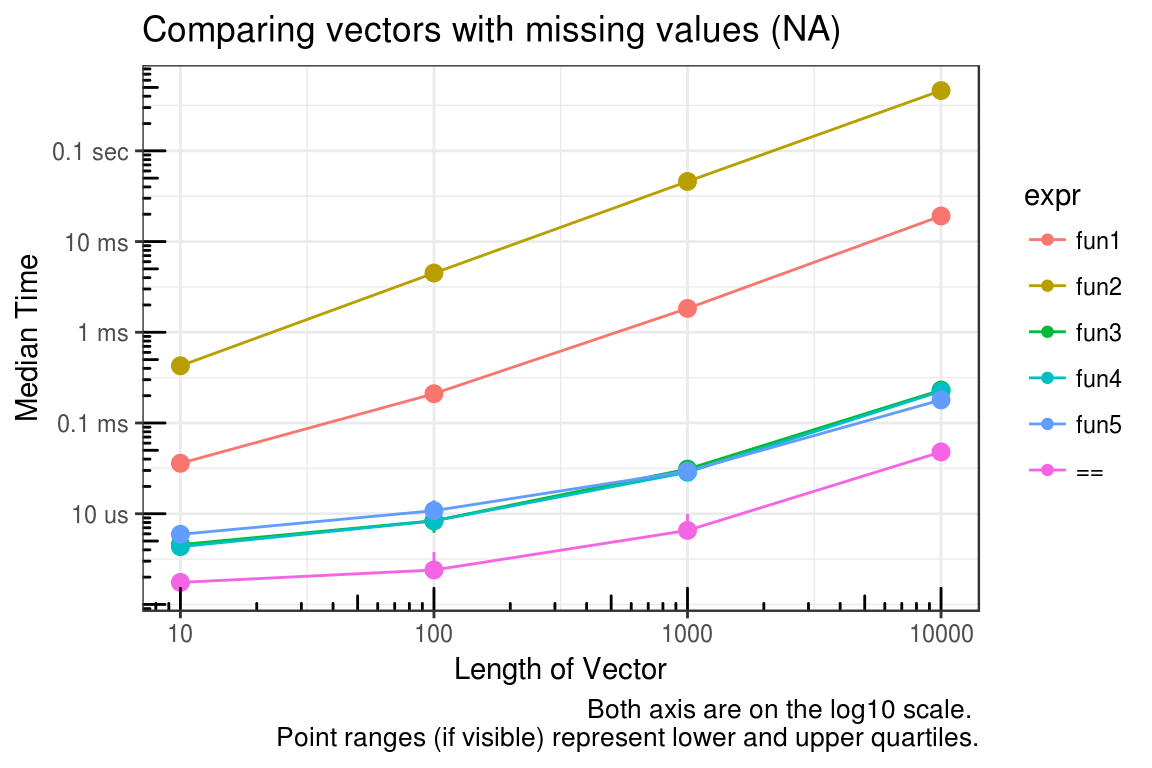You can see that looping element by element comparisons is very slow (which is to be expected in R), but modifying the output from `==` is acceptable.

## `[` – Subsetting with logical and integer vectors

Subsetting with integer vectors is faster than logical vectors.

``````# Simulate data
vec_sizes <- 10^(1:6)
test_list <- lapply(vec_sizes, function(x) {
sample(10L, size = x, replace = TRUE)
})
test_list_log <- lapply(test_list, function(x) {x > 5})
test_list_int <- lapply(test_list, function(x) {which(x > 5)})
test_functions <- alist(
logical = test_list[[x]][test_list_log[[x]]],
integer = test_list[[x]][test_list_int[[x]]]
)

# Run benchmark
res <- mb_summary(
data_list = list(
"test_list" = test_list,
"test_list_log" = test_list_log,
"test_list_int" = test_list_int
),
func_list  = test_functions,
size = vec_sizes,
times = 30,
unit = "s",
check = TRUE
)

# Plot results
ggplot(data = res, mapping = aes(x = size, y = median, color = expr)) +
geom_line() +
geom_point() +
geom_pointrange(aes(ymin = lq, ymax = uq, color = expr), show.legend = FALSE) +
scale_x_continuous(trans = "log10", breaks = vec_sizes, labels = vec_sizes) +
scale_y_continuous(trans = "log10", breaks = log_breaks(res), labels = time_conversion(log_breaks(res))) +
labs(
title = "Subsetting a vector (~50% dropped)",
x = "Length of Vector",
y = "Median Time",
caption = "Both axis are on the log10 scale. \nPoint ranges (if visible) represent lower and upper quartiles."
) +
annotation_logticks() +
theme_bw()``````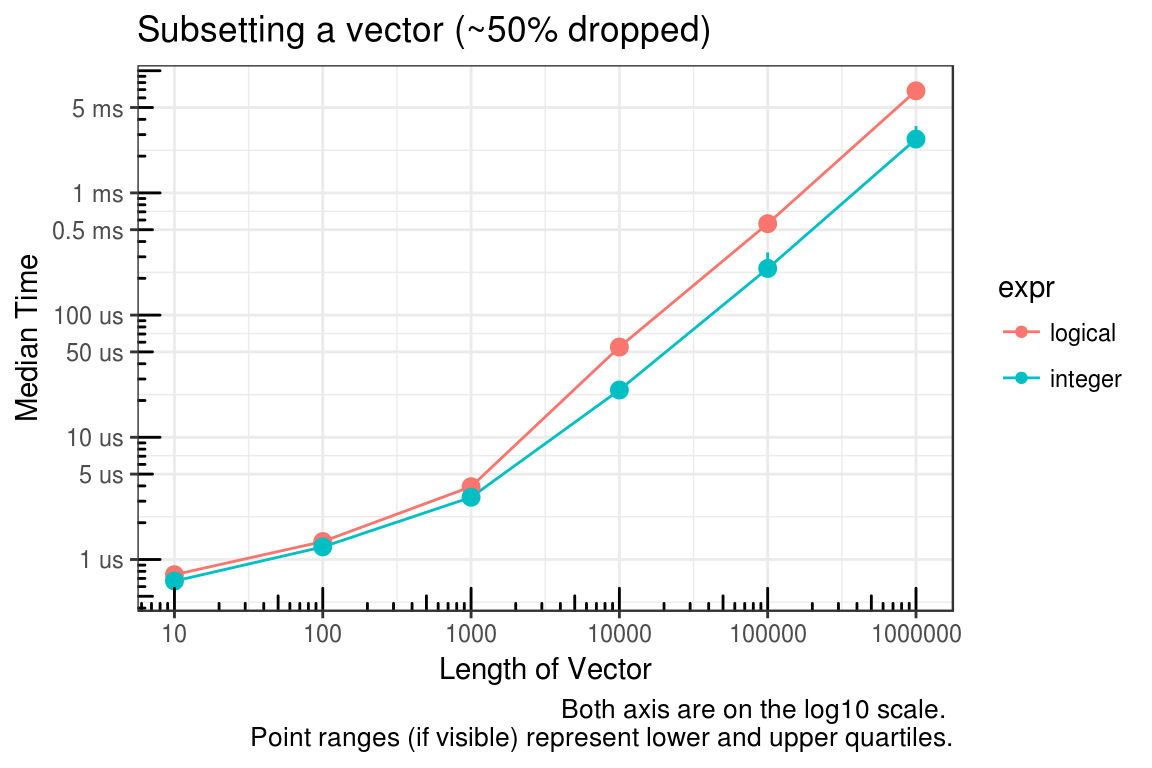The logical vector, by definition, must be the same size as the vector to be subsetted and the integer vector can be much smaller. The plot above is for the case where approximately 50% of the vector is being subsetted (dropped). We’ll check this again for approximately 10% and 90%.

``````# Simulate data for 10% dropped
test_list_log <- lapply(test_list, function(x) {x > 1})
test_list_int <- lapply(test_list, function(x) {which(x > 1)})

# Run benchmark
res <- mb_summary(
data_list = list(
"test_list" = test_list,
"test_list_log" = test_list_log,
"test_list_int" = test_list_int
),
func_list  = test_functions,
size = vec_sizes,
times = 30,
unit = "s",
check = TRUE
)

# Plot results
ggplot(data = res, mapping = aes(x = size, y = median, color = expr)) +
geom_line() +
geom_point() +
geom_pointrange(aes(ymin = lq, ymax = uq, color = expr), show.legend = FALSE) +
scale_x_continuous(trans = "log10", breaks = vec_sizes, labels = vec_sizes) +
scale_y_continuous(trans = "log10", breaks = log_breaks(res), labels = time_conversion(log_breaks(res))) +
labs(
title = "Subsetting a vector (~10% dropped)",
x = "Length of Vector",
y = "Median Time",
caption = "Both axis are on the log10 scale. \nPoint ranges (if visible) represent lower and upper quartiles."
) +
annotation_logticks() +
theme_bw()``````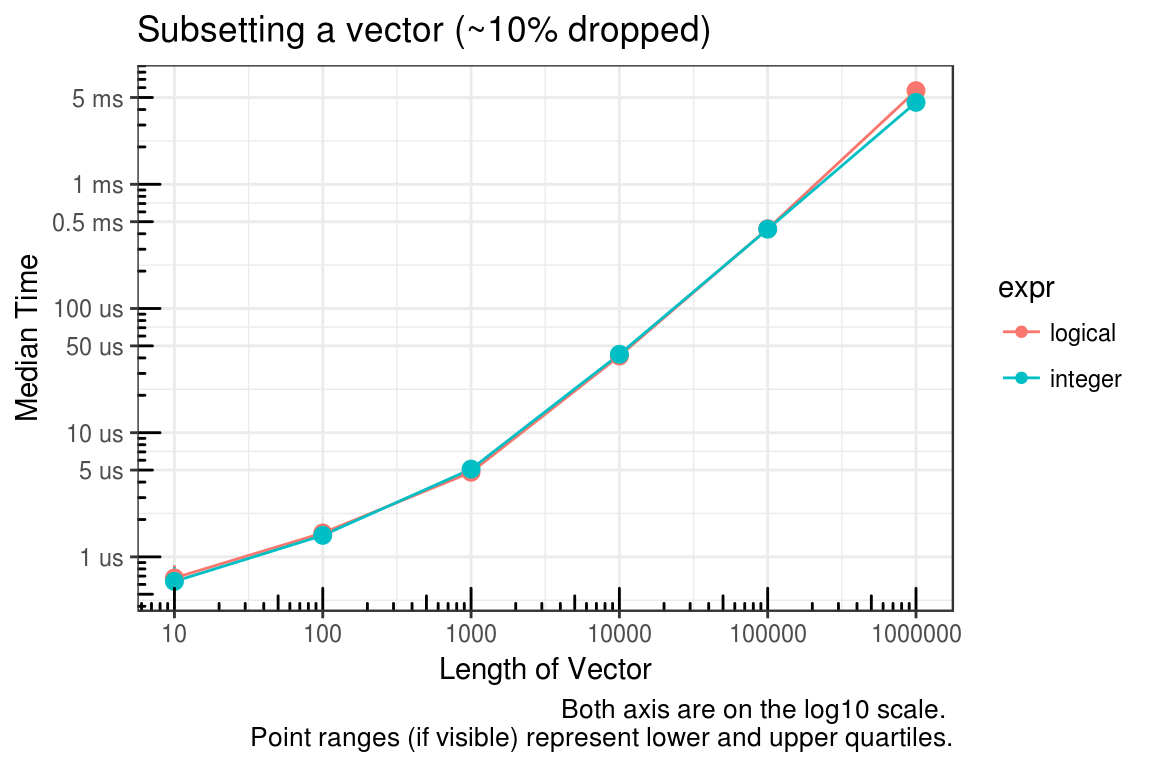``````# Simulate data for 90% dropped
test_list_log <- lapply(test_list, function(x) {x > 9})
test_list_int <- lapply(test_list, function(x) {which(x > 9)})

# Run benchmark
res <- mb_summary(
data_list = list(
"test_list" = test_list,
"test_list_log" = test_list_log,
"test_list_int" = test_list_int
),
func_list  = test_functions,
size = vec_sizes,
times = 30,
unit = "s",
check = TRUE
)

# Plot results
ggplot(data = res, mapping = aes(x = size, y = median, color = expr)) +
geom_line() +
geom_point() +
geom_pointrange(aes(ymin = lq, ymax = uq, color = expr), show.legend = FALSE) +
scale_x_continuous(trans = "log10", breaks = vec_sizes, labels = vec_sizes) +
scale_y_continuous(trans = "log10", breaks = log_breaks(res), labels = time_conversion(log_breaks(res))) +
labs(
title = "Subsetting a vector (~90% dropped)",
x = "Length of Vector",
y = "Median Time",
caption = "Both axis are on the log10 scale. \nPoint ranges (if visible) represent lower and upper quartiles."
) +
annotation_logticks() +
theme_bw()``````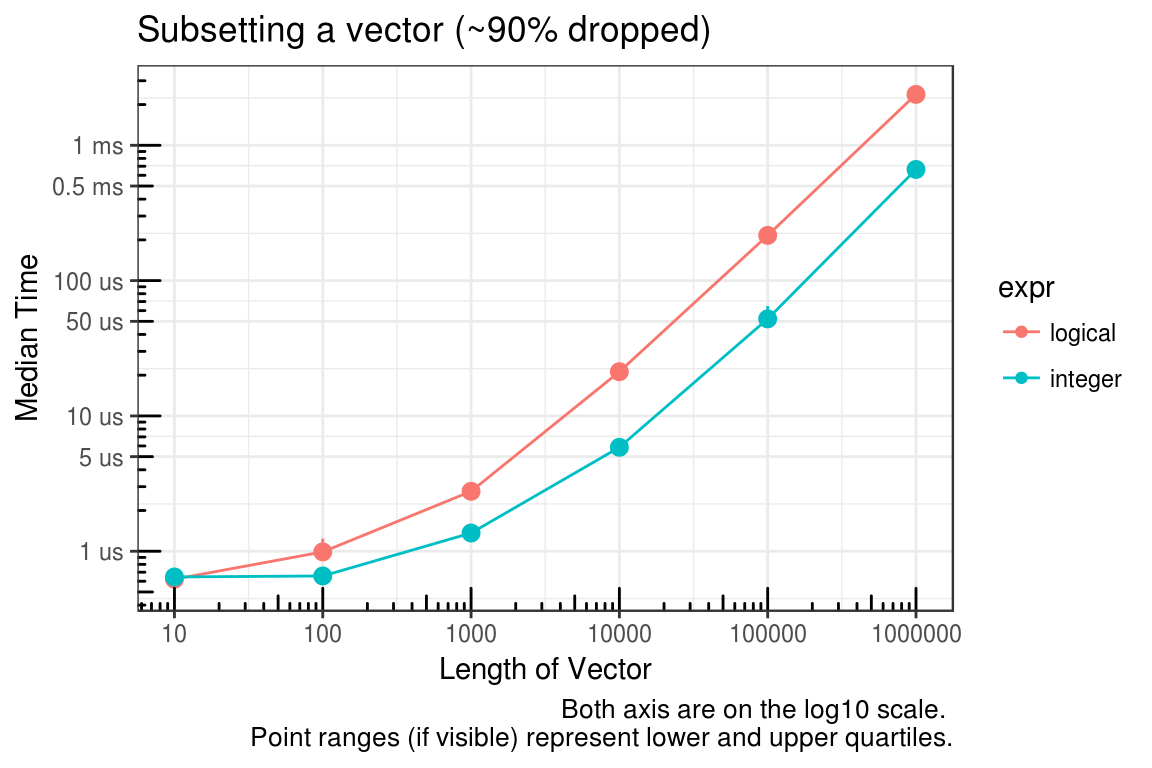So it appears the main culprit is the size of the vector used to subset. Since an integer vector will often be smaller than a logical vector, using integers seems to be the logical choice :).

Next, you might say what about `which`? The time to convert the logical vector to integer was not considered in the previous benchmarks. As seen below, this is a valid concern.

``````# Simulate data for 10% dropped
test_list_log <- lapply(test_list, function(x) {x > 1})
test_list_int <- lapply(test_list, function(x) {which(x > 1)})
test_functions <- alist(
logical = test_list[[x]][test_list_log[[x]]],
`which(logical)` = test_list[[x]][which(test_list_log[[x]])]
)

# Run benchmark
res <- mb_summary(
data_list = list(
"test_list" = test_list,
"test_list_log" = test_list_log,
"test_list_int" = test_list_int
),
func_list  = test_functions,
size = vec_sizes,
times = 30,
unit = "s",
check = TRUE
)

# Plot results
ggplot(data = res, mapping = aes(x = size, y = median, color = expr)) +
geom_line() +
geom_point() +
geom_pointrange(aes(ymin = lq, ymax = uq, color = expr), show.legend = FALSE) +
scale_x_continuous(trans = "log10", breaks = vec_sizes, labels = vec_sizes) +
scale_y_continuous(trans = "log10", breaks = log_breaks(res), labels = time_conversion(log_breaks(res))) +
labs(
title = "Subsetting a vector (~10% dropped)",
x = "Length of Vector",
y = "Median Time",
caption = "Both axis are on the log10 scale. \nPoint ranges (if visible) represent lower and upper quartiles."
) +
annotation_logticks() +
theme_bw()``````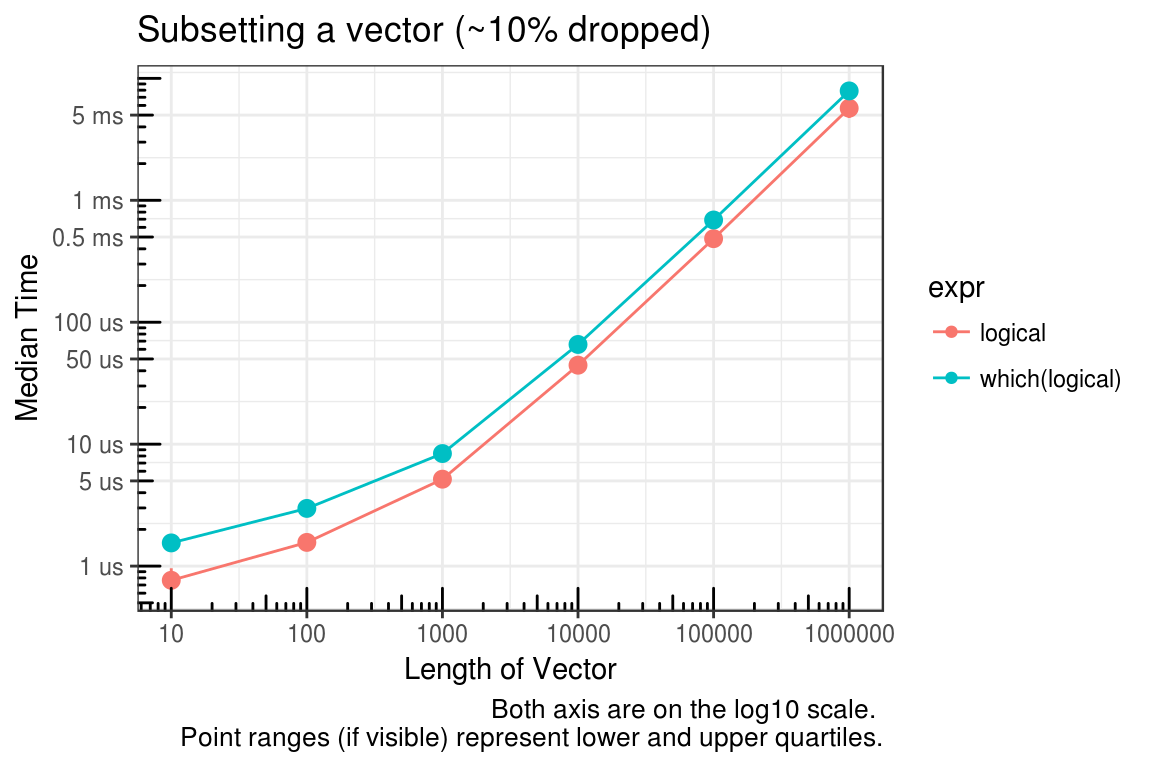``````# Simulate data for 90% dropped
test_list_log <- lapply(test_list, function(x) {x > 9})
test_list_int <- lapply(test_list, function(x) {which(x > 9)})
test_functions <- alist(
logical = test_list[[x]][test_list_log[[x]]],
`which(logical)` = test_list[[x]][which(test_list_log[[x]])]
)

# Run benchmark
res <- mb_summary(
data_list = list(
"test_list" = test_list,
"test_list_log" = test_list_log,
"test_list_int" = test_list_int
),
func_list  = test_functions,
size = vec_sizes,
times = 30,
unit = "s",
check = TRUE
)

# Plot results
ggplot(data = res, mapping = aes(x = size, y = median, color = expr)) +
geom_line() +
geom_point() +
geom_pointrange(aes(ymin = lq, ymax = uq, color = expr), show.legend = FALSE) +
scale_x_continuous(trans = "log10", breaks = vec_sizes, labels = vec_sizes) +
scale_y_continuous(trans = "log10", breaks = log_breaks(res), labels = time_conversion(log_breaks(res))) +
labs(
title = "Subsetting a vector (~90% dropped)",
x = "Length of Vector",
y = "Median Time",
caption = "Both axis are on the log10 scale. \nPoint ranges (if visible) represent lower and upper quartiles."
) +
annotation_logticks() +
theme_bw()``````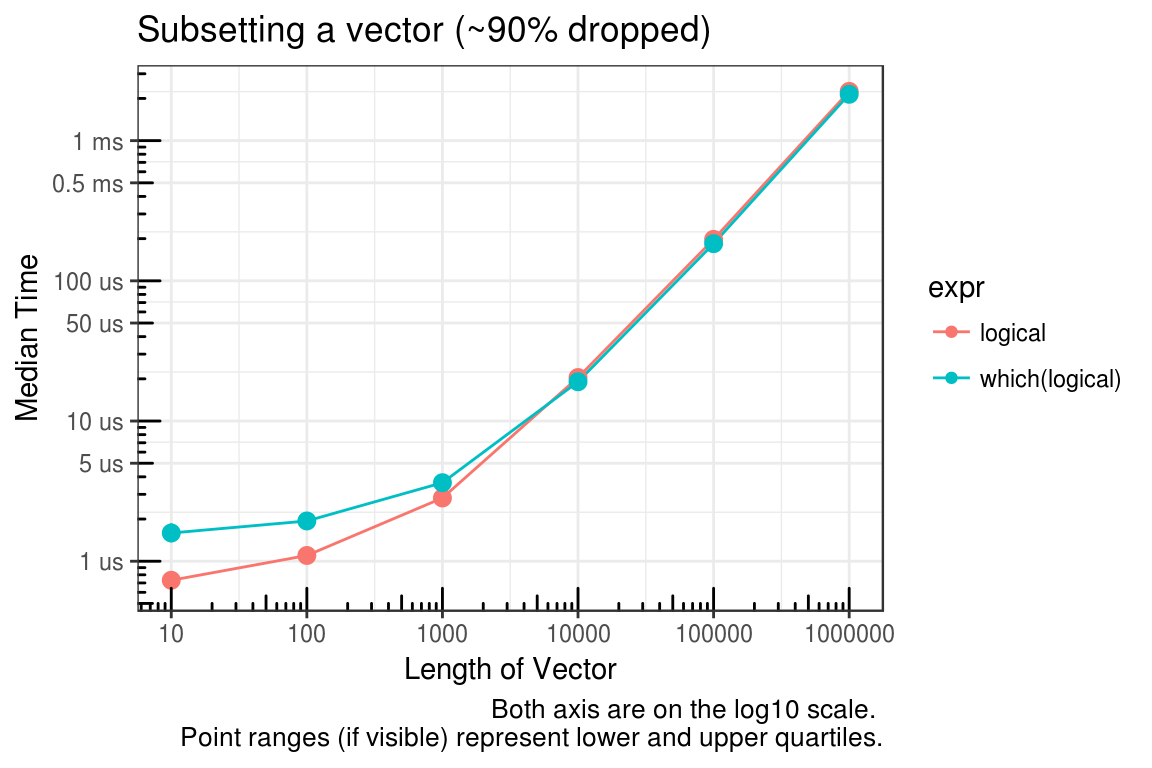In summary, if you are able to pre-allocate the subset indices, then use integers. If you cannot pre-allocate then sticking with logical indices may make sense.

## `as.numeric` – Convert a factor that is actually numeric

This comes straight from the `?factor` documentation.

To transform a factor f to approximately its original numeric values, as.numeric(levels(f))[f] is recommended and slightly more efficient than as.numeric(as.character(f)).

We are only concerned about non-integer cases because `as.integer` handles factors as you would expect. If you need to convert numeric values or handle both cases (integer or numeric) then the two solutions to this problem are `as.numeric(as.character(f))`, which is usually easy to remember, and `as.numeric(levels(f))[f]`, which is hard to remember. One interesting note is that the R FAQ provides a slightly different “hard” solution (`as.numeric(levels(f))[as.integer(f)]`) than found in the factor documentation.

The relative speed between these functions depends on the number of values in the levels of the factor. The ‘hard’ methods will convert a factor with a small number of levels faster than the easy method. If the number of levels is the same as the size of the factor, then all methods will be similar in speed.

These methods only work if the factor vector has levels/labels that are numeric.

``````# Simulate data
vec_sizes <- 10^(1:6)
## Make sure NA are handled correctly below
test_list <- lapply(vec_sizes, function(x) {
factor(sample(c(rnorm(9), NA), x, replace = TRUE))
})
test_functions <- alist(
hard_faq = as.numeric(levels(test_list[[x]]))[as.integer(test_list[[x]])],
hard_doc = as.numeric(levels(test_list[[x]]))[test_list[[x]]],
easy_doc = as.numeric(as.character(test_list[[x]]))
)

# Run benchmark
res <- mb_summary(
data_list = list("test_list" = test_list),
func_list  = test_functions,
size = vec_sizes,
times = 30,
unit = "s",
check = TRUE
)

# Plot results
ggplot(data = res, mapping = aes(x = size, y = median, color = expr)) +
geom_line() +
geom_point() +
geom_pointrange(aes(ymin = lq, ymax = uq, color = expr), show.legend = FALSE) +
scale_x_continuous(trans = "log10", breaks = vec_sizes, labels = vec_sizes) +
scale_y_continuous(trans = "log10", breaks = log_breaks(res), labels = time_conversion(log_breaks(res))) +
labs(
title = "Converting a factor that is actually numeric (Level length ~9)",
x = "Length of factor vector",
y = "Median Time",
caption = "Both axis are on the log10 scale. \nPoint ranges (if visible) represent lower and upper quartiles."
) +
annotation_logticks() +
theme_bw()``````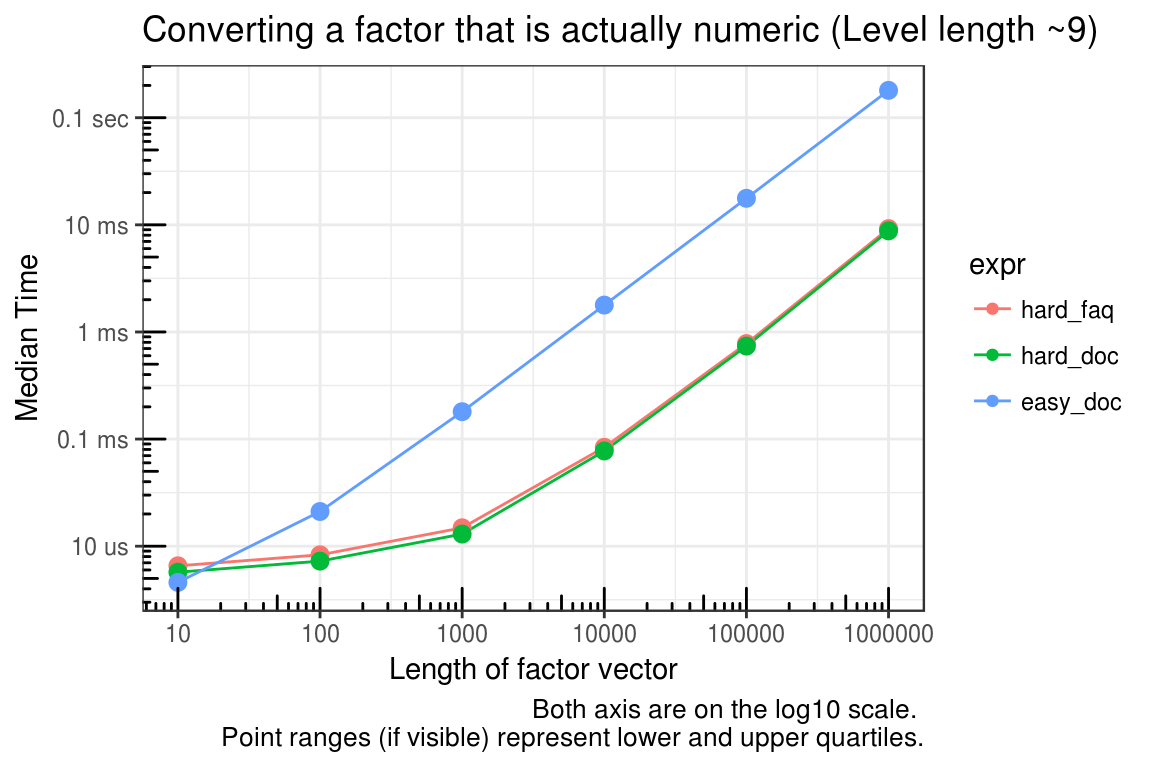``````# Simulate data
vec_sizes <- 10^(1:6)
## Make sure NA are handled correctly below
test_list <- lapply(vec_sizes, function(x) {
factor(c(rnorm(x), NA))
})
test_functions <- alist(
hard_faq = as.numeric(levels(test_list[[x]]))[as.integer(test_list[[x]])],
hard_doc = as.numeric(levels(test_list[[x]]))[test_list[[x]]],
easy_doc = as.numeric(as.character(test_list[[x]]))
)

# Run benchmark
res <- mb_summary(
data_list = list("test_list" = test_list),
func_list  = test_functions,
size = vec_sizes,
times = 30,
unit = "s",
check = TRUE
)

# Plot results
ggplot(data = res, mapping = aes(x = size, y = median, color = expr)) +
geom_line() +
geom_point() +
geom_pointrange(aes(ymin = lq, ymax = uq, color = expr), show.legend = FALSE) +
scale_x_continuous(trans = "log10", breaks = vec_sizes, labels = vec_sizes) +
scale_y_continuous(trans = "log10", breaks = log_breaks(res), labels = time_conversion(log_breaks(res))) +
labs(
title = "Converting a factor that is actually numeric (Level length ~N)",
x = "Length of factor vector",
y = "Median Time",
caption = "Both axis are on the log10 scale. \nPoint ranges (if visible) represent lower and upper quartiles."
) +
annotation_logticks() +
theme_bw()``````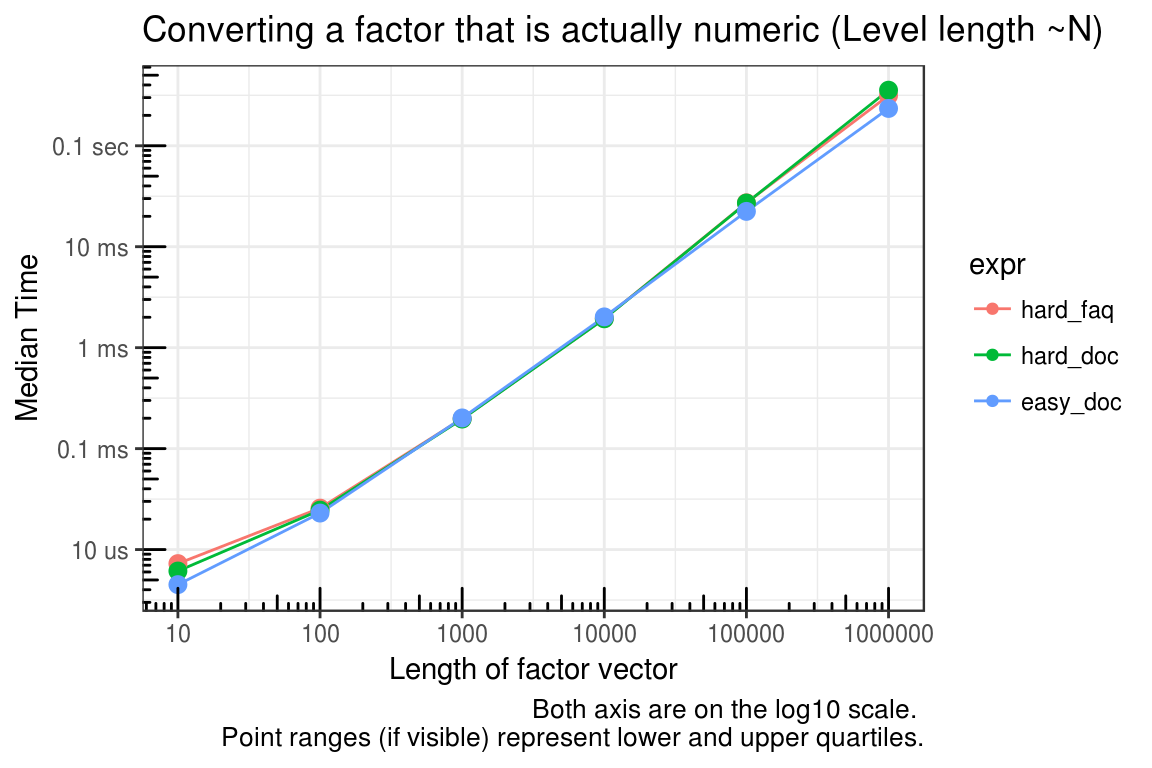`as.integer` is the only choice that makes sense if you have strictly integer levels.

``````f <- factor(sample(1:9, 100, replace = TRUE))
mb <- microbenchmark(
as.integer = as.integer(f),
hard_faq = as.integer(levels(f))[as.integer(f)],
hard_doc = as.integer(levels(f))[f],
easy_doc = as.integer(as.character(f)),
check = check_equality,
unit = "relative"
)
knitr::kable(
summary(mb)[c("expr", "lq", "median", "uq", "neval")],
format = "html",
align = c("r", rep('l', 4)),
digits = 1,
caption = paste("Unit:", mb_units(mb)),
table.attr='class="pure-table table-responsive"'
)``````
Table 1: Unit: relative
expr lq median uq neval
as.integer 1.0 1.0 1.0 100
hard_faq 5.6 5.1 4.8 100
hard_doc 4.7 4.3 4.0 100
easy_doc 14.9 13.3 12.4 100

## `lengths` – Get lengths along a list

`length` is a fast primitive function, but when finding the length of many objects along a list, `lengths` (notice the ending `s`) is better than applying `length` over the list.

``````# Simulate data
vec_sizes <- 10^(1:5)
test_list <- lapply(vec_sizes, function(x) {
replicate(
x,
sample(10L, size = sample(10L, 1), replace = TRUE),
simplify = FALSE
)
})
test_functions <- alist(
lengths = lengths(test_list[[x]]),
vapply = vapply(
X = test_list[[x]],
FUN = length,
FUN.VALUE = integer(1),
USE.NAMES = FALSE
)
)

# Run benchmark
res <- mb_summary(
data_list = list("test_list" = test_list),
func_list  = test_functions,
size = vec_sizes,
times = 30,
unit = "s",
check = TRUE
)

# Plot results
ggplot(data = res, mapping = aes(x = size, y = median, color = expr)) +
geom_line() +
geom_point() +
geom_pointrange(aes(ymin = lq, ymax = uq, color = expr), show.legend = FALSE) +
scale_x_continuous(trans = "log10", breaks = vec_sizes, labels = vec_sizes) +
scale_y_continuous(trans = "log10", breaks = log_breaks(res), labels = time_conversion(log_breaks(res))) +
labs(
title = "Returning the length of list items",
x = "Length of List",
y = "Median Time",
caption = "Both axis are on the log10 scale. \nPoint ranges (if visible) represent lower and upper quartiles."
) +
annotation_logticks() +
theme_bw()``````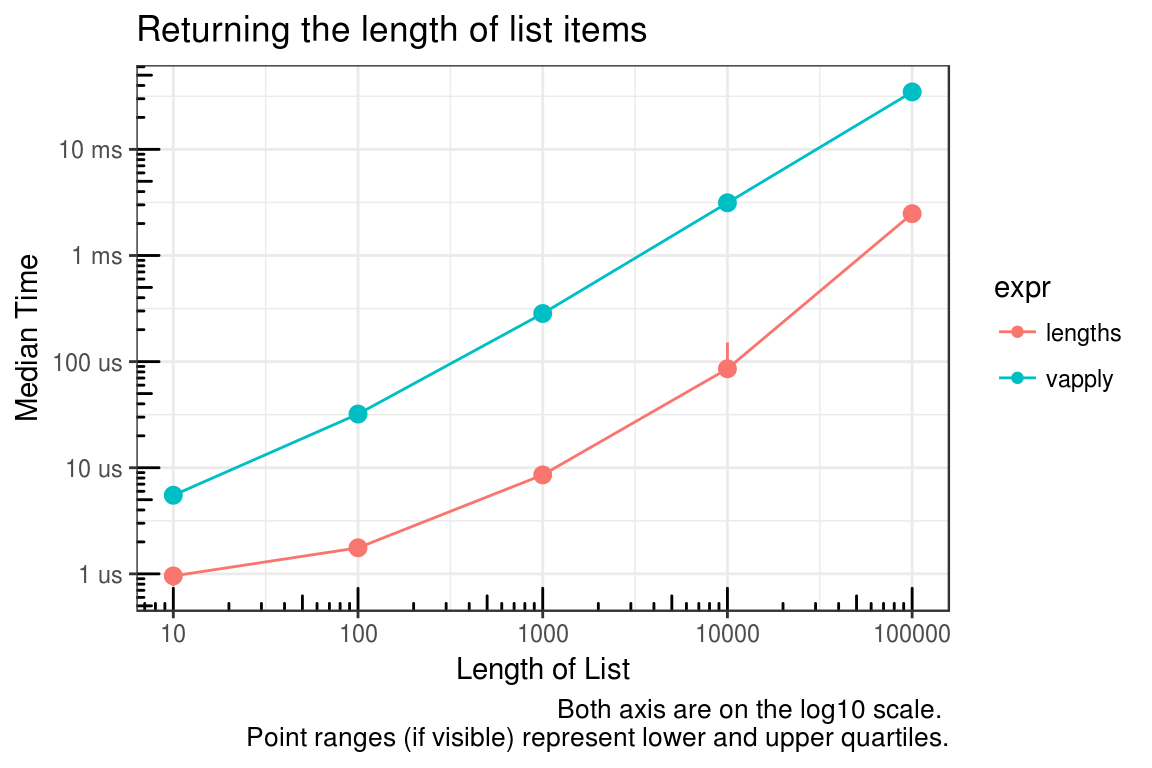Microbenchmark summary for lengths of 1 and 100000.

``````mb1 <- microbenchmark(
lengths = lengths(list(1)),
vapply = vapply(
X = list(1),
FUN = length,
FUN.VALUE = integer(1),
USE.NAMES = FALSE
),
times = 30,
check = check_equality
)
knitr::kable(
summary(mb1)[c("expr", "lq", "median", "uq", "neval")],
format = "html",
align = c("r", rep('l', 4)),
digits = 1,
caption = paste("Unit:", mb_units(mb1)),
table.attr='class="pure-table table-responsive"'
)``````
Table 2: Unit: nanoseconds
expr lq median uq neval
lengths 539 676.0 791 30
vapply 2724 2881.5 3026 30
``````mb2 <- microbenchmark(
lengths = lengths(test_list[[length(vec_sizes)]]),
vapply = vapply(
X = test_list[[length(vec_sizes)]],
FUN = length,
FUN.VALUE = integer(1),
USE.NAMES = FALSE
),
times = 30,
check = check_equality
)
knitr::kable(
summary(mb2)[c("expr", "lq", "median", "uq", "neval")],
format = "html",
align = c("r", rep('l', 4)),
digits = 1,
caption = paste("Unit:", mb_units(mb2)),
table.attr='class="pure-table table-responsive"'
)``````
Table 3: Unit: milliseconds
expr lq median uq neval
lengths 2.4 2.5 2.6 30
vapply 34.3 34.8 35.8 30

## `nrow` – Get data.frame row lengths

Here we compare the methods of getting the number of rows in a data.frame. Consider the penalty for cases of applying the function many times versus storing and calling the saved object.

• stored_value: The time it takes to get the length which has already been assigned to an object.
• stored_subset1: The time it takes to get the length from the object returned by `dim` using single bracket (`[`) indexing.
• stored_subset2: as above using double bracket (`[[`) indexing.
• internal: Using the internal function `.row_names_info`. Note that although this is an internal function with a dot name, it does appear to be safe to use being that it is a generic exported function.
• primitive: using the primitive function `dim` along with single bracket (`[`) indexing.
• convenience: using the convenience function `nrow` (which just calls `dim()[1L]`).

The benchmark below has values for the simplest possible case.

``````# Simulate test data
data <- data.frame(a = 1)
dims <- dim(data)
n_row <- dims[1L]

# Benchmark methods
mb <- microbenchmark(
stored_value = n_row,
stored_subset1 = dims[1L],
stored_subset2 = dims[[1L]],
internal = .row_names_info(data, type = 2L),
primitive = dim(data)[1L],
convenience = nrow(data)
)
knitr::kable(
summary(mb)[c("expr", "lq", "median", "uq", "neval")],
format = "html",
align = c("r", rep('l', 4)),
digits = 1,
caption = paste("Unit:", mb_units(mb)),
table.attr='class="pure-table table-responsive"'
)``````
Table 4: Unit: nanoseconds
expr lq median uq neval
stored_value 50.5 64.0 72.0 100
stored_subset1 131.5 181.0 209.5 100
stored_subset2 193.0 259.0 289.0 100
internal 490.5 545.0 632.5 100
primitive 2657.5 2800.5 3020.5 100
convenience 2700.0 2829.0 2966.0 100

The following graph provides a summary of actually applying these methods to a list of dataframes.

``````# Simulate data
vec_sizes <- 10^(1:5)
## Note that the structure function below is just dput() output for
## data.frame(a=1), but is much faster for replicating.
test_list <- lapply(vec_sizes, function(x) {
replicate(
x,
structure(list(a = 1), .Names = "a", row.names = c(NA, -1L), class = "data.frame"),
simplify = FALSE
)
})
test_functions <- alist(
stored_subset1 = lapply(test_list[[x]], function(y) {dims}),
internal = lapply(test_list[[x]], function(y) {.row_names_info(y, type = 2L)}),
primitive = lapply(test_list[[x]], function(y) {dim(y)[1L]}),
convenience = lapply(test_list[[x]], function(y) {nrow(y)})
)

# Run benchmark
res <- mb_summary(
data_list = list("test_list" = test_list),
func_list  = test_functions,
size = vec_sizes,
times = 30,
unit = "s",
check = TRUE
)

# Plot results
ggplot(data = res, mapping = aes(x = size, y = median, color = expr)) +
geom_line() +
geom_point() +
geom_pointrange(aes(ymin = lq, ymax = uq, color = expr), show.legend = FALSE) +
scale_x_continuous(trans = "log10", breaks = vec_sizes, labels = vec_sizes) +
scale_y_continuous(trans = "log10", breaks = log_breaks(res), labels = time_conversion(log_breaks(res))) +
labs(
title = "Returning number of rows in a data.frame",
x = "Number of data.frames",
y = "Median Time",
caption = "Both axis are on the log10 scale. \nPoint ranges (if visible) represent lower and upper quartiles."
) +
annotation_logticks() +
theme_bw()``````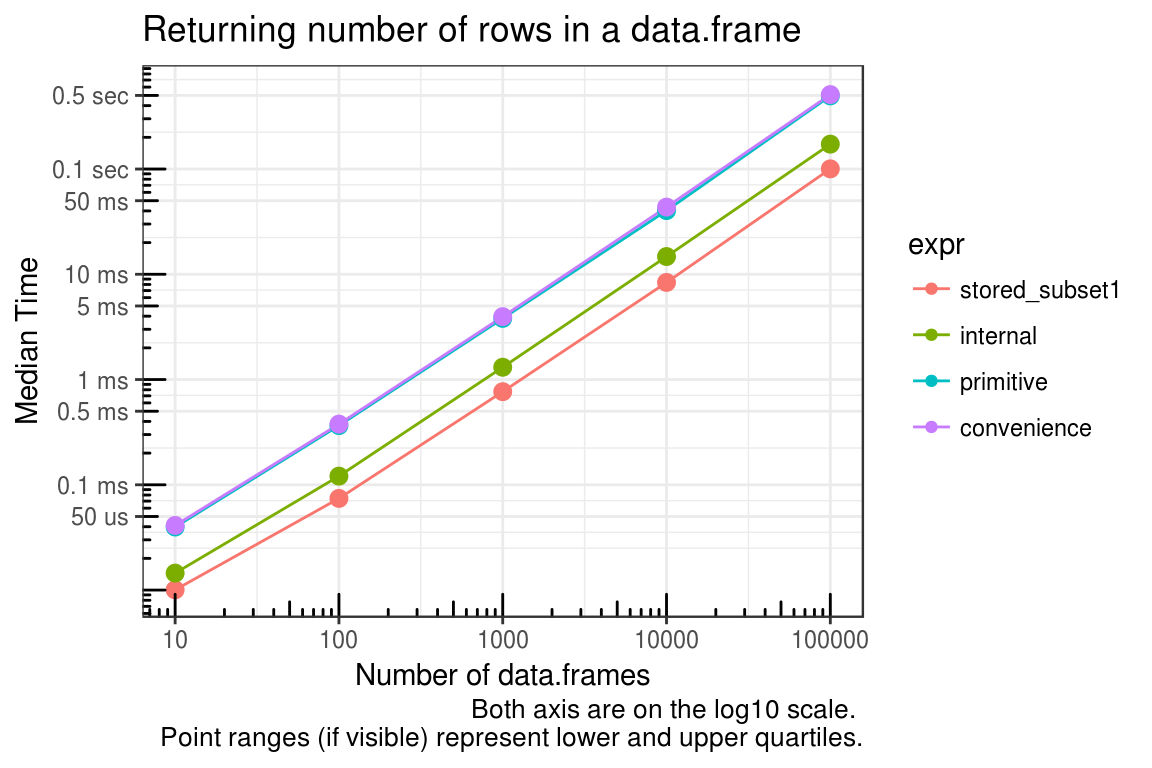Last updated 2018-04-15